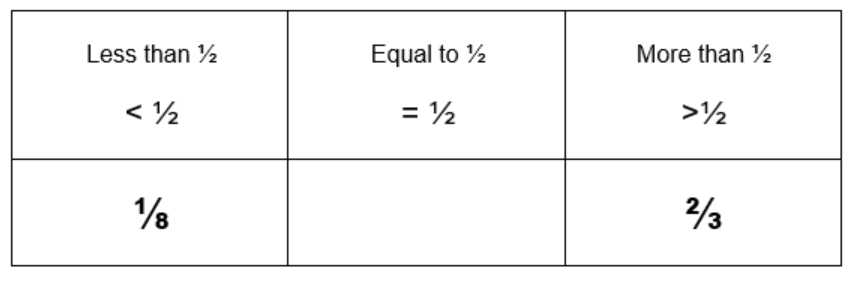Learning Objective:
Identify fractions as more than or less than ½ (or a given amount).

Intended Outcome:
Accurate identification of fractions, comparing faction values.

Game Objective:
To collect 5 fractions more than ½ and 5 fractions less than ½.

Materials:

• 2x ten-sided dice
• Paper and pen for recording

Instructions:
Play this game in pairs or small groups.

1. Players take turns to roll two ten-sided dice.
2. The smaller number is the numerator and the larger number is the denominator.
3. Place the fraction made on a chart showing if it is more than, less than or equal to ½.
For example, if the numbers 8 and 1 were rolled, the fraction would be ⅛. Or, if the two numbers rolled were 2 and 3, the fraction would be ⅔.4. The first player to have 5 fractions in the less than ½ column AND 5 fractions in the more than ½ column wins.

Hint: A fraction wall could be a useful tool!

Variations:

Change the given fraction to another, for example instead of finding fractions less than and more than ½ find fractions that are less than and more than ⅓.

Increase the amount of fractions the student must collect to win.

Use decimal notation instead of fraction notation.

Recommended Posts